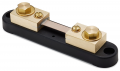# Measuring Dc current greater than device max

#### cgrams18

Joined Sep 20, 2023
4
Hello, I'm looking into a 48VDC 6killowatt system drawing around 117A. My questions is how to monitor the voltage through a 60 volt max 30milliamp acquisition system. I was wondering the method to measure the voltage without inducing the current to the measurement device? Thank you.

#### MrAl

Joined Jun 17, 2014
10,891
Hello, I'm looking into a 48VDC 6killowatt system drawing around 117A. My questions is how to monitor the voltage through a 60 volt max 30milliamp acquisition system. I was wondering the method to measure the voltage without inducing the current to the measurement device? Thank you.
Not sure what you mean exactly, but to measure higher voltage you use a voltage divider and to measure higher current you can use different methods.
One of the methods used for current meters that can not go up to the full current is to add a shunt resistance. The shunt resistance value is calculated to bypass some of the current so that the meter reads a scaled version of the actual current.
For example, and this is just a example, if you had a 1 amp current meter with internal resistance of 1 Ohm and you needed to measure 10 amps, then if you connect a shunt resistor with value 1/9 Ohms (approximately 0.111 Ohms) then when the current is 10 amps the meter reads 1 amp, so you multiply the reading on the meter by 10 in your head. The shunt resistor must be able to handle the power too though at the max current.

To calculate the shunt resistor value you just use the idea of two resistors in parallel and equating the two currents and resistances. The end result is:
Rs=(Rint*I1)/(I2-I1)

where
Rint is the internal resistance of the meter,
I1 is the max current of the meter,
I2 is the higher current level to be measured,
Rs is the shunt resistor value, to be connected in parallel to the meter.

Fs=I2/I1

The power of Rs has to be high enough so it does not burn up. The power is (I2-I1)^2*Rs or approximately I2^2*Rs. You should get a resistor that is rated for at least twice this value or P=2*Rs*I2^2.

This method has been around for years but you do have to be careful about the power rating of the added resistor and make sure the connections are tight or can end up with big errors in the readings. It also helps to test it with a known current before you use it for anything.

If this is about voltage instead of current, then you use a voltage divider and multiply the readings by the scale factor of the voltage divider. You also have to make sure the meter does not load the voltage divider too much.

#### Ian0

Joined Aug 7, 2020
8,940
If you are only monitoring the voltage, why does the current matter?

#### cgrams18

Joined Sep 20, 2023
4
If you are only monitoring the voltage, why does the current matter?
My concern was just the current through the circuit damaging the voltage measurement device. The circuit is nearly 120A while the device has a max of 30mA

#### Ian0

Joined Aug 7, 2020
8,940
My concern was just the current through the circuit damaging the voltage measurement device. The circuit is nearly 120A while the device has a max of 30mA
How is the device connected? Does it have an input, output and 0V terminals?

#### cgrams18

Joined Sep 20, 2023
4
How is the device connected? Does it have an input, output and 0V terminals?
It would be in parallel with the device and the voltage would ve an input to monitor.

#### Ian0

Joined Aug 7, 2020
8,940
It would be in parallel with the device and the voltage would ve an input to monitor.
In which case, how could the current possibly go through it and cause a problem?

#### cgrams18

Joined Sep 20, 2023
4
In which case, how could the current possibly go through it and cause a problem?
Forgive me I'm newer at this and was just looking to understand prior to potentially damaging equipment.

#### Ian0

Joined Aug 7, 2020
8,940
Forgive me I'm newer at this and was just looking to understand prior to potentially damaging equipment.
Post some more details about the device you are connecting. Photos, perhaps.

#### Alec_t

Joined Sep 17, 2013
14,005

#### ronsimpson

Joined Oct 7, 2019
2,795
Measuring 48V on a 60V system is good.
We need to know more about you 30mA input. Pictures, model number, schematic, anything.

We often measure very small volts across a shunt resistor. If you get a 0.001 ohm resistor and put 117A through it the voltage across the resistor will be 0.117 volts.

It is likely that you 30mA input has a shunt resistor but the wrong value for your use. Maybe it has a 1 ohm resistor. By putting a much lower resistor across the input we probably can trick the 30mA input into being a 300A input.

Here is a picture of an old style shunt. The 100A passes from large bolt to large bolt. The voltage meter looks across the small screws.